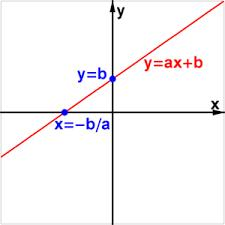# Specify 5462

P (x) = 15x- (5x + 10,000)

specify x so that P (x) = 0

x =  1000

### Step-by-step explanation:

15x-(5x+10000)=0

15·x-(5·x+10000)=0

10x = 10000

x = 10000/10 = 1000

x = 1000

Our simple equation calculator calculates it.Did you find an error or inaccuracy? Feel free to write us. Thank you!

Tips for related online calculators
Do you have a linear equation or system of equations and looking for its solution? Or do you have a quadratic equation?